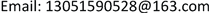1. 引言

2. 数独问题的多项式表示

1) 如上图1设出16个变量 x 1 , x 2 , ⋯ , x 16 ，分别表示16个格位，用有限域 F 11 中的四个元素 { 1 , 2 , 3 , 4 } 分别表示要填的四个数字(注：选择在有限域 F 11 中进行计算，是因为我们所应用的计算软件Singular分别用 { − p − 1 2 , − 1 , 0 , 1 , ⋯ , p − 1 2 } 这11个元素来表示 F p (其中p为素数)中个元素， p = 11 是使 F p 包含数字1，2，3，4的最小的素数了，为了读写方便，所以选择在 F 11 中进行计算。)；

2) 写出表示格位取值的16个多项式： F ( x i ) = ∏ n = 1 4 ( x i − n ) ,   ( i = 1 , 2 , ⋯ , 16 ) ；

3) 若格位 x i 和格位 x j 处不能填相同的数字，则用 G ( x i , x j ) : = F ( x i ) − F ( x j ) x i − x j 来表示，这样的方程共有56个，分别用来表示每行、每列、每宫内的格位中所填数字不能相同。

3. 吴特征列与不可约特征序列

2) r > t ，而且对于每个 j ≤ t 都有 T j ~ S j 成立。如果 S ≺ T ，那么称 T 的秩高于 S 的秩，记为 T ≻ S 。如果 T ≻ S 和 T ≺ S 都不成立，那么称 T 和 S 具有相同的秩，记为 T ~ S 。将 T ≺ S 或者 T ~ S 简记为 T ≾ S 。并规定平凡升列的秩总是低于非平凡升列的秩。

P 的基列可以通过如下步骤找出：设 F 1 : = P ，令 B 1 是 F 1 中秩最低的多项式。如果 B 1 是非零常数，那么 [ B 1 ] 就是 P 的一个基列。否则，令 F 2 : = { F ∈ F 1 \ B 1 : F 对 B 1 是 约 化 的 } 。如果 F 2 = ∅ ，那么 [ B 1 ] 就是

P 的一个基列。否则，设 B 2 是 F 2 中秩最低的多项式。易知 B 1 ≺ B 2 。可以类似地依次构造 F 3 , F 4 , ⋯ ，因为 P 是非空有限的多项式集合，而基列或是一个非零常数，或是作为一个三角列最多含有n个多项式，所以上述构造过程必定会在有限步之后终止，最后我们就可以得到 P 的一个基列。

P 的吴特征列的算法如下：1) 设 F : = P ， R : = P ；2) 如果集合 R 非空，则求取 R 的一个基列 C ；3) 若 C 中含有 K 中的元素，则另 R ∶ = ∅ ，否则另 R : = { p r e m ( F , C ) : F ∈ F \ C } \ { 0 } ；4) 另 F : = P ∪ C ∪ R ，并返回第2)步，直到集合 R 为空集为止。最后一次求出的基列 C 即为多项式组 P 的吴特征列。该算法的终止性及正确性可参见  。

T = [ T 1 ( x 1 , ⋯ , x p 1 ) , T 2 ( x 1 , ⋯ , x p 1 , ⋯ , x p 2 ) , ⋯ , T r ( x 1 , ⋯ , x p 1 , ⋯ , x p 2 , ⋯ , x p r ) ] ,

4. 应用及结论